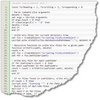# Use DOS Batch to show last month date with the date commandUse a DOS script to automate a process where by using the date /T command would return the previous month, rather than the current month. This actually sounds easier than it is. For the desired results, a couple of conditions were considered. Firstly, if in the month of January or one, then the subtracted value of one minus one equals zero. A month that does not exist. Therefore, if the month equals zero set it to 12. Another obvious condition is that if the month equals 12, then the year must also be subtracted by one. A couple of other hurdles, that were not readily considered is having to pad the value of 1 through 9 with a 0. The date /T command already pads 1 through 9, however, when passing through the set /a command and switch, the padded value became a single digit. However, the date value of 08 or 09 could not pass through the set /a command with switch without throwing an error. The error has to do with octal notation values. The remedy is to set the for 08 and 09 to 8 and 9 prior to passing through the set /a command and switch. The result is the following code.

```:: Format the date
for /f "tokens=2,3,4 delims=/ " %%I in ('DATE /T') do ( set yyyy=%%K
set mm=%%I )
:: Corrects Invalid number error for the set command for octal notation.
if %mm%==08 set mm=8
if %mm%==09 set mm=9
:: Set month to last month
set /a mm=%mm%-1
:: If subtracting one month equals zero then reset to 12
if %mm%==0 set mm=12
:: If month equals 12 then subtract one year
if %mm%==12 set /a yyyy=%yyyy%-1
:: If month is less than 10 then pad with a zero
if %mm% LSS 10 set mm=0%mm%

@echo Month %mm% Year %yyyy%```

Alternately to using the following command the date can be disected like this %date:~10,4%_%date:~4,2%_%date:~7,2%

```for /f "tokens=2,3,4 delims=/ " %%I in ('DATE /T') do ( set yyyy=%%K
set mm=%%I )```